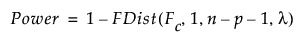Publication date: 05/24/2021

## Power for a Single Parameter

This section describes how power for the test of a single parameter is computed. Use the following notation:

X

The model matrix. See Coding for Nominal Effects in Fitting Linear Models for information on the coding for nominal effects. Also, See Model Matrix.

Note: You can view the model matrix by running Fit Model. Then select Save Columns > Save Coding Table from the red triangle menu for the main report.

βι

The parameter corresponding to the term of interest.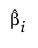The least squares estimator of βi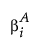The Anticipated Coefficient value. The difference you want to detect is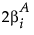.

The variance of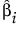is given by the ith diagonal entry of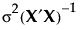, where σ2 is the error variance. Denote the ith diagonal entry of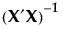by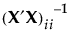.

The error variance, σ2, is estimated by the MSE, and has n p 1 degrees of freedom, where n is the number of observations and p is the number of terms other than the intercept in the model. If n p 1 = 0, then JMP sets the degrees of freedom for the error to 1. This allows the power to be estimated for parameters in a saturated design.

The test of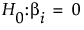is given by: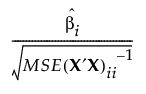or equivalently by: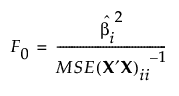Under the null hypothesis, the test statistic F0 has an F distribution on 1 and n p 1 degrees of freedom.

If the true value of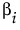is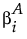, then F0 has a noncentral F distribution with noncentrality parameter given by: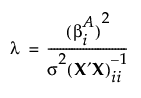To compute the power of the test, first solve for the α-level critical value Fc: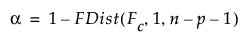Then calculate the power as follows: